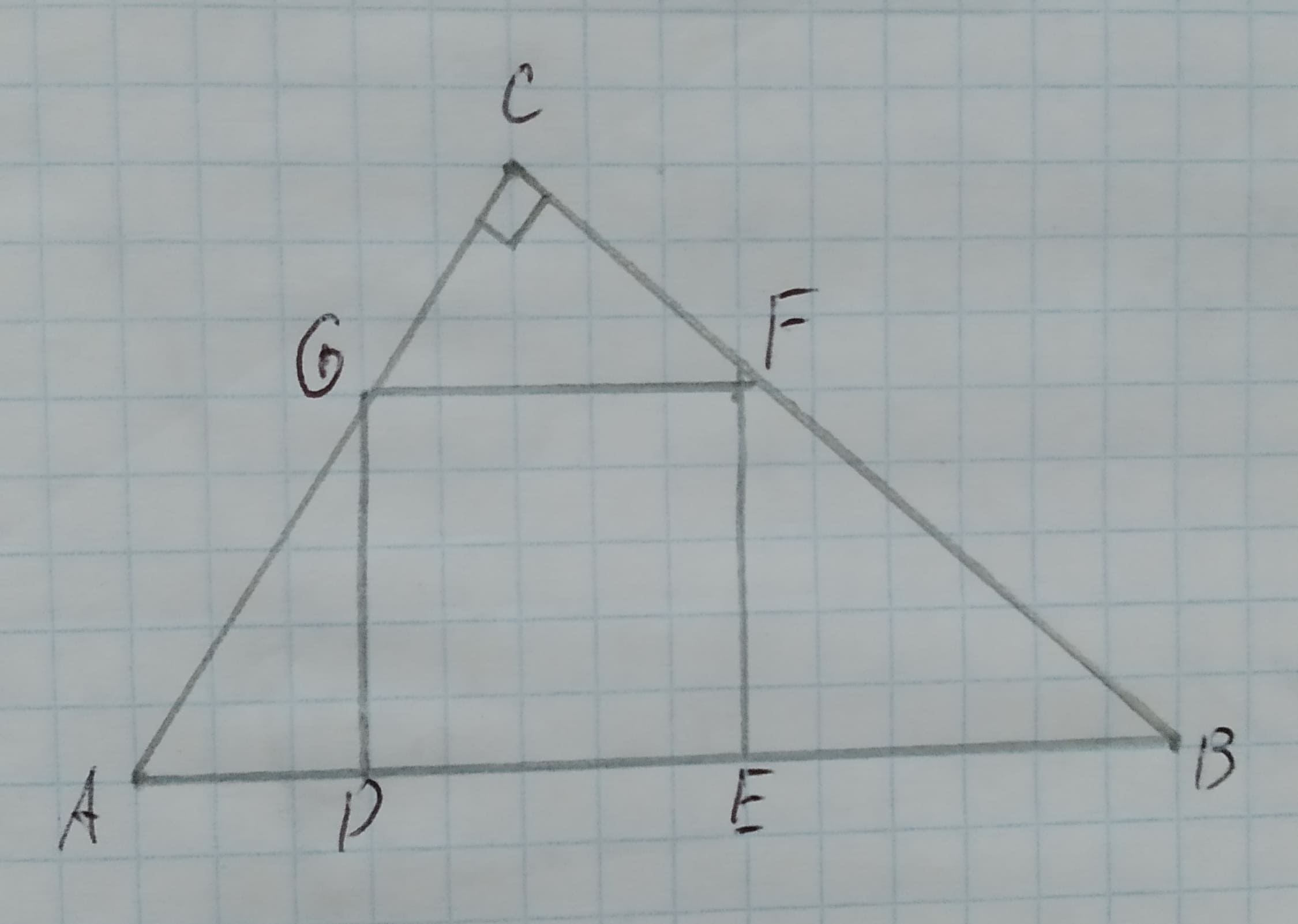# Proving Similarity in the figure DEFG is a square. Prove the following:/_ADG~/_FEBGiven:The given figure is,12210202691.jpgbeljuA 2020-11-08 Answered

Proving Similarity in the figure DEFG is a square. Prove the following:
$\mathrm{△}ADG\sim \mathrm{△}FEB$
Given:
The given figure is,You can still ask an expert for help

• Questions are typically answered in as fast as 30 minutes

Solve your problem for the price of one coffee

• Math expert for every subject
• Pay only if we can solve itSabadisO
Approach:
If the first triangle is similar to the second triangle and second triangle is similar to the third triangle then, the first triangle is similar to the third triangle.
Calculation:
Its given that DEFG is a square.
Consider $\mathrm{△}GCF\phantom{\rule{1em}{0ex}}\text{and}\phantom{\rule{1em}{0ex}}\mathrm{△}FEB$.
$\mathrm{\angle }FEB=\mathrm{\angle }GCF$ both are ${90}^{\circ }$.
And $GF\mid \mid AB,thus,\mathrm{\angle }CFG=\mathrm{\angle }FBE$ as they are alternate angles.
Therefore, $\mathrm{△}GCF\sim \mathrm{△}FEB$ by AA rule.
Since, $\mathrm{△}GCF\sim \mathrm{△}FEB\phantom{\rule{1em}{0ex}}\text{and}\phantom{\rule{1em}{0ex}}\mathrm{△}ADG\sim \mathrm{△}GCF,hence,\mathrm{△}ADG\sim \mathrm{△}FEB$
Conclusion:
Hence, it is proved that $\mathrm{△}ADG\sim \mathrm{△}FEB$.